x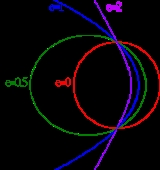Orbital eccentricityOverview

The orbital eccentricity of an astronomical body is the amount by which its orbit deviates from a perfect circle
Circle
A circle is a simple shape of Euclidean geometry consisting of those points in a plane that are a given distance from a given point, the centre. The distance between any of the points and the centre is called the radius....

, where 0 is perfectly circular, and 1.0 is a parabola
Parabola
In mathematics, the parabola is a conic section, the intersection of a right circular conical surface and a plane parallel to a generating straight line of that surface...

, and no longer a closed orbit. It derives its name from the parameters of conic sections
Conic section
In mathematics, a conic section is a curve obtained by intersecting a cone with a plane. In analytic geometry, a conic may be defined as a plane algebraic curve of degree 2...

, as every Kepler orbit
Kepler orbit
In celestial mechanics, a Kepler orbit describes the motion of an orbiting body as an ellipse, parabola, or hyperbola, which forms a two-dimensional orbital plane in three-dimensional space...

is a conic section.
In a two-body problem
Two-body problem
In classical mechanics, the two-body problem is to determine the motion of two point particles that interact only with each other. Common examples include a satellite orbiting a planet, a planet orbiting a star, two stars orbiting each other , and a classical electron orbiting an atomic nucleus In...

with inverse-square-law force, every orbit
Orbit
In physics, an orbit is the gravitationally curved path of an object around a point in space, for example the orbit of a planet around the center of a star system, such as the Solar System...

is a Kepler orbit. The eccentricity
Eccentricity (mathematics)
In mathematics, the eccentricity, denoted e or \varepsilon, is a parameter associated with every conic section. It can be thought of as a measure of how much the conic section deviates from being circular.In particular,...

of this Kepler orbit
Kepler orbit
In celestial mechanics, a Kepler orbit describes the motion of an orbiting body as an ellipse, parabola, or hyperbola, which forms a two-dimensional orbital plane in three-dimensional space...

is a positive number that defines its shape.

The eccentricity may take the following values:
• circular orbit
Circular orbit
A circular orbit is the orbit at a fixed distance around any point by an object rotating around a fixed axis.Below we consider a circular orbit in astrodynamics or celestial mechanics under standard assumptions...

: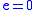• elliptic orbit
Elliptic orbit
In astrodynamics or celestial mechanics an elliptic orbit is a Kepler orbit with the eccentricity less than 1; this includes the special case of a circular orbit, with eccentricity equal to zero. In a stricter sense, it is a Kepler orbit with the eccentricity greater than 0 and less than 1 . In a...

: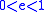(see Ellipse
Ellipse
In geometry, an ellipse is a plane curve that results from the intersection of a cone by a plane in a way that produces a closed curve. Circles are special cases of ellipses, obtained when the cutting plane is orthogonal to the cone's axis...

)
• parabolic trajectory
Parabolic trajectory
In astrodynamics or celestial mechanics a parabolic trajectory is a Kepler orbit with the eccentricity equal to 1. When moving away from the source it is called an escape orbit, otherwise a capture orbit...

: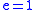(see Parabola
Parabola
In mathematics, the parabola is a conic section, the intersection of a right circular conical surface and a plane parallel to a generating straight line of that surface...

)
• hyperbolic trajectory
Hyperbolic trajectory
In astrodynamics or celestial mechanics a hyperbolic trajectory is a Kepler orbit with the eccentricity greater than 1. Under standard assumptions a body traveling along this trajectory will coast to infinity, arriving there with hyperbolic excess velocity relative to the central body. Similarly to...

: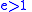(see Hyperbola
Hyperbola
In mathematics a hyperbola is a curve, specifically a smooth curve that lies in a plane, which can be defined either by its geometric properties or by the kinds of equations for which it is the solution set. A hyperbola has two pieces, called connected components or branches, which are mirror...

)

The eccentricityis given by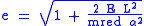where E is the total orbital energy,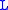is the angular momentum
Angular momentum
In physics, angular momentum, moment of momentum, or rotational momentum is a conserved vector quantity that can be used to describe the overall state of a physical system...

,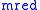is the reduced mass
Reduced mass
Reduced mass is the "effective" inertial mass appearing in the two-body problem of Newtonian mechanics. This is a quantity with the unit of mass, which allows the two-body problem to be solved as if it were a one-body problem. Note however that the mass determining the gravitational force is not...

.Encyclopedia
The orbital eccentricity of an astronomical body is the amount by which its orbit deviates from a perfect circle
Circle
A circle is a simple shape of Euclidean geometry consisting of those points in a plane that are a given distance from a given point, the centre. The distance between any of the points and the centre is called the radius....

, where 0 is perfectly circular, and 1.0 is a parabola
Parabola
In mathematics, the parabola is a conic section, the intersection of a right circular conical surface and a plane parallel to a generating straight line of that surface...

, and no longer a closed orbit. It derives its name from the parameters of conic sections
Conic section
In mathematics, a conic section is a curve obtained by intersecting a cone with a plane. In analytic geometry, a conic may be defined as a plane algebraic curve of degree 2...

, as every Kepler orbit
Kepler orbit
In celestial mechanics, a Kepler orbit describes the motion of an orbiting body as an ellipse, parabola, or hyperbola, which forms a two-dimensional orbital plane in three-dimensional space...

is a conic section.

## Definition

In a two-body problem
Two-body problem
In classical mechanics, the two-body problem is to determine the motion of two point particles that interact only with each other. Common examples include a satellite orbiting a planet, a planet orbiting a star, two stars orbiting each other , and a classical electron orbiting an atomic nucleus In...

with inverse-square-law force, every orbit
Orbit
In physics, an orbit is the gravitationally curved path of an object around a point in space, for example the orbit of a planet around the center of a star system, such as the Solar System...

is a Kepler orbit. The eccentricity
Eccentricity (mathematics)
In mathematics, the eccentricity, denoted e or \varepsilon, is a parameter associated with every conic section. It can be thought of as a measure of how much the conic section deviates from being circular.In particular,...

of this Kepler orbit
Kepler orbit
In celestial mechanics, a Kepler orbit describes the motion of an orbiting body as an ellipse, parabola, or hyperbola, which forms a two-dimensional orbital plane in three-dimensional space...

is a positive number that defines its shape.

The eccentricity may take the following values:
• circular orbit
Circular orbit
A circular orbit is the orbit at a fixed distance around any point by an object rotating around a fixed axis.Below we consider a circular orbit in astrodynamics or celestial mechanics under standard assumptions...

:• elliptic orbit
Elliptic orbit
In astrodynamics or celestial mechanics an elliptic orbit is a Kepler orbit with the eccentricity less than 1; this includes the special case of a circular orbit, with eccentricity equal to zero. In a stricter sense, it is a Kepler orbit with the eccentricity greater than 0 and less than 1 . In a...

:(see Ellipse
Ellipse
In geometry, an ellipse is a plane curve that results from the intersection of a cone by a plane in a way that produces a closed curve. Circles are special cases of ellipses, obtained when the cutting plane is orthogonal to the cone's axis...

)
• parabolic trajectory
Parabolic trajectory
In astrodynamics or celestial mechanics a parabolic trajectory is a Kepler orbit with the eccentricity equal to 1. When moving away from the source it is called an escape orbit, otherwise a capture orbit...

:(see Parabola
Parabola
In mathematics, the parabola is a conic section, the intersection of a right circular conical surface and a plane parallel to a generating straight line of that surface...

)
• hyperbolic trajectory
Hyperbolic trajectory
In astrodynamics or celestial mechanics a hyperbolic trajectory is a Kepler orbit with the eccentricity greater than 1. Under standard assumptions a body traveling along this trajectory will coast to infinity, arriving there with hyperbolic excess velocity relative to the central body. Similarly to...

:(see Hyperbola
Hyperbola
In mathematics a hyperbola is a curve, specifically a smooth curve that lies in a plane, which can be defined either by its geometric properties or by the kinds of equations for which it is the solution set. A hyperbola has two pieces, called connected components or branches, which are mirror...

)

The eccentricityis given bywhere E is the total orbital energy,is the angular momentum
Angular momentum
In physics, angular momentum, moment of momentum, or rotational momentum is a conserved vector quantity that can be used to describe the overall state of a physical system...

,is the reduced mass
Reduced mass
Reduced mass is the "effective" inertial mass appearing in the two-body problem of Newtonian mechanics. This is a quantity with the unit of mass, which allows the two-body problem to be solved as if it were a one-body problem. Note however that the mass determining the gravitational force is not...

. andthe coefficient of the inverse-square law central force such as gravity or electrostatics
Electrostatics
Electrostatics is the branch of physics that deals with the phenomena and properties of stationary or slow-moving electric charges....

in classical physics
Classical physics
What "classical physics" refers to depends on the context. When discussing special relativity, it refers to the Newtonian physics which preceded relativity, i.e. the branches of physics based on principles developed before the rise of relativity and quantum mechanics...

: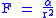(is negative for an attractive force, positive for a repulsive one) (see also Kepler problem
Kepler problem
In classical mechanics, the Kepler problem is a special case of the two-body problem, in which the two bodies interact by a central force F that varies in strength as the inverse square of the distance r between them. The force may be either attractive or repulsive...

).

or in the case of a gravitational force: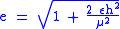whereis the specific orbital energy
Specific orbital energy
In the gravitational two-body problem, the specific orbital energy \epsilon\,\! of two orbiting bodies is the constant sum of their mutual potential energy and their total kinetic energy , divided by the reduced mass...

(total energy divided by the reduced mass),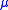the standard gravitational parameter
Standard gravitational parameter
In astrodynamics, the standard gravitational parameter μ of a celestial body is the product of the gravitational constant G and the mass M of the body.\mu=GM \ The SI units of the standard gravitational parameter are m3s−2....

based on the total mass, and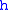the specific relative angular momentum
Specific relative angular momentum
The specific relative angular momentum is also known as the areal momentum .In astrodynamics, the specific relative angular momentum of two orbiting bodies is the vector product of the relative position and the relative velocity. Equivalently, it is the total angular momentum divided by the...

(angular momentum
Angular momentum
In physics, angular momentum, moment of momentum, or rotational momentum is a conserved vector quantity that can be used to describe the overall state of a physical system...

divided by the reduced mass).

For values of e from 0 to 1 the orbit's shape is an increasingly elongated (or flatter) ellipse; for values of e from 1 to infinity the orbit is a hyperbola branch making a total turn of 2 arccsc e, decreasing from 180 to 0 degrees. The limit case between an ellipse and a hyperbola is for e precisely 1 which is parabola.

Radial trajectories are classified as elliptic, parabolic, or hyperbolic based on the energy of the orbit, not the eccentricity. Radial orbits have zero angular momentum and hence eccentricity equal to one. Keeping the energy constant and reducing the angular momentum, elliptic, parabolic, and hyperbolic orbits each tend to the corresponding type of radial trajectory while e tends to 1 (or in the parabolic case: remains 1).

For a repulsive force only the hyperbolic trajectory, including the radial version, is applicable.

For elliptical orbits, a simple proof shows that arcsin() yields the projection angle of a perfect circle to an ellipse
Ellipse
In geometry, an ellipse is a plane curve that results from the intersection of a cone by a plane in a way that produces a closed curve. Circles are special cases of ellipses, obtained when the cutting plane is orthogonal to the cone's axis...

of eccentricity. For example, to view the eccentricity of the planet Mercury (=0.2056), one must simply calculate the inverse sine to find the projection angle of 11.86 degrees. Next, tilt any circular object (such as a coffee mug viewed from the top) by that angle and the apparent ellipse
Ellipse
In geometry, an ellipse is a plane curve that results from the intersection of a cone by a plane in a way that produces a closed curve. Circles are special cases of ellipses, obtained when the cutting plane is orthogonal to the cone's axis...

projected to your eye will be of that same eccentricity.

## Etymology

From Medieval Latin eccentricus, derived from Greek ekkentros "out of the center", from ek-, ex- "out of" + kentron "center". Eccentric first appeared in English in 1551, with the definition "a circle in which the earth, sun. etc. deviates from its center." Five years later, in 1556, an adjective form of the word was added.

## Calculation

Eccentricity of an orbit
Orbit
In physics, an orbit is the gravitationally curved path of an object around a point in space, for example the orbit of a planet around the center of a star system, such as the Solar System...

can be calculated from orbital state vectors
Orbital state vectors
In astrodynamics or celestial dynamics orbital state vectors are vectors of position and velocity that together with their time uniquely determine the state of an orbiting body....

as a magnitude
Magnitude (mathematics)
The magnitude of an object in mathematics is its size: a property by which it can be compared as larger or smaller than other objects of the same kind; in technical terms, an ordering of the class of objects to which it belongs....

of eccentricity vector
Eccentricity vector
In astrodynamics, the eccentricity vector of a Kepler orbit is the vector pointing towards the periapsis having a magnitude equal to the orbit's scalar eccentricity. The magnitude is unitless. For Kepler orbits the eccentricity vector is a constant of motion...

: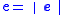where:
•is eccentricity vector
Eccentricity vector
In astrodynamics, the eccentricity vector of a Kepler orbit is the vector pointing towards the periapsis having a magnitude equal to the orbit's scalar eccentricity. The magnitude is unitless. For Kepler orbits the eccentricity vector is a constant of motion...

.

For elliptical orbits it can also be calculated from distance at apoapsis and periapsis: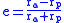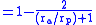where:
•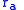is radius at apoapsis (i.e., the farthest distance of the orbit to the center of mass
Center of mass
In physics, the center of mass or barycenter of a system is the average location of all of its mass. In the case of a rigid body, the position of the center of mass is fixed in relation to the body...

of the system, which is a focus
Focus (geometry)
In geometry, the foci are a pair of special points with reference to which any of a variety of curves is constructed. For example, foci can be used in defining conic sections, the four types of which are the circle, ellipse, parabola, and hyperbola...

of the ellipse).
•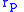is radius at periapsis (the closest distance).

The eccentricity of an elliptical orbit can also be used to obtain the ratio of the periapsis to the apoapsis: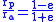## Examples

The eccentricity of the Earth
Earth
Earth is the third planet from the Sun, and the densest and fifth-largest of the eight planets in the Solar System. It is also the largest of the Solar System's four terrestrial planets...

's orbit is currently about 0.0167, meaning that the Earth's orbit is nearly circular. Over hundreds of thousands of years, the eccentricity of the Earth's orbit varies from nearly 0.0034 to almost 0.058 as a result of gravitational attractions among the planets (see graph).

In other values, Mercury
Mercury (planet)
Mercury is the innermost and smallest planet in the Solar System, orbiting the Sun once every 87.969 Earth days. The orbit of Mercury has the highest eccentricity of all the Solar System planets, and it has the smallest axial tilt. It completes three rotations about its axis for every two orbits...

(with an eccentricity of 0.2056) holds the title as the largest value among the planets of the Solar System. Prior to the redefinition of its planetary status, the dwarf planet Pluto
Pluto
Pluto, formal designation 134340 Pluto, is the second-most-massive known dwarf planet in the Solar System and the tenth-most-massive body observed directly orbiting the Sun...

held this title with an eccentricity of about 0.248. The Moon
Moon
The Moon is Earth's only known natural satellite,There are a number of near-Earth asteroids including 3753 Cruithne that are co-orbital with Earth: their orbits bring them close to Earth for periods of time but then alter in the long term . These are quasi-satellites and not true moons. For more...

also holds a notable value at 0.0549. For the values for all planets in one table, see List of gravitationally rounded objects of the Solar System.

Most of the solar system's asteroid
Asteroid
Asteroids are a class of small Solar System bodies in orbit around the Sun. They have also been called planetoids, especially the larger ones...

s have eccentricities between 0 and 0.35 with an average value of 0.17. Their comparatively high eccentricities are probably due to the influence of Jupiter
Jupiter
Jupiter is the fifth planet from the Sun and the largest planet within the Solar System. It is a gas giant with mass one-thousandth that of the Sun but is two and a half times the mass of all the other planets in our Solar System combined. Jupiter is classified as a gas giant along with Saturn,...

and to past collisions.

The eccentricity of comet
Comet
A comet is an icy small Solar System body that, when close enough to the Sun, displays a visible coma and sometimes also a tail. These phenomena are both due to the effects of solar radiation and the solar wind upon the nucleus of the comet...

s is most often close to 1. Periodic comets have highly eccentric elliptical orbits, with eccentricities just below 1; Halley's Comet's elliptical orbit, for example, has a value of 0.967. Non-periodic comets follow near-parabolic orbits and thus have eccentricities very close to 1. Examples include Comet Hale-Bopp
Comet Hale-Bopp
Comet Hale–Bopp was perhaps the most widely observed comet of the 20th century, and one of the brightest seen for many decades...

with a value of 0.995 and comet C/2006 P1 (McNaught) with a value of 1.000019. As Hale-Bopp's value is less than 1, its orbit is elliptical and so the comet will in fact return. Comet McNaught has a hyperbolic orbit while within the influence of the planets, but is still bound to the Sun with an orbital period of about 100,000 years. As of a 2010 Epoch
Epoch (astronomy)
In astronomy, an epoch is a moment in time used as a reference point for some time-varying astronomical quantity, such as celestial coordinates, or elliptical orbital elements of a celestial body, where these are subject to perturbations and vary with time...

, Comet C/1980 E1
C/1980 E1
C/1980 E1 is a non-periodic comet discovered by Edward L. G. Bowell on February 11, 1980. C/1980 E1 is leaving the solar system on a hyperbolic trajectory with a higher velocity than any other object ever observed....

has the largest eccentricity of any known hyperbolic comet with an eccentricity of 1.057, and will leave the solar system
Solar System
The Solar System consists of the Sun and the astronomical objects gravitationally bound in orbit around it, all of which formed from the collapse of a giant molecular cloud approximately 4.6 billion years ago. The vast majority of the system's mass is in the Sun...

indefinitely.

Planet Neptune
Neptune
Neptune is the eighth and farthest planet from the Sun in the Solar System. Named for the Roman god of the sea, it is the fourth-largest planet by diameter and the third largest by mass. Neptune is 17 times the mass of Earth and is slightly more massive than its near-twin Uranus, which is 15 times...

's largest moon Triton
Triton (moon)
Triton is the largest moon of the planet Neptune, discovered on October 10, 1846, by English astronomer William Lassell. It is the only large moon in the Solar System with a retrograde orbit, which is an orbit in the opposite direction to its planet's rotation. At 2,700 km in diameter, it is...

has an eccentricity of 0.000016 which is the smallest eccentricity of any known body in the solar system; its orbit is as close to a perfect circle as can be currently measured.

## Mean eccentricity

The mean eccentricity of an object is the average eccentricity as a result of perturbations
Perturbation (astronomy)
Perturbation is a term used in astronomy in connection with descriptions of the complex motion of a massive body which is subject to appreciable gravitational effects from more than one other massive body....

over a given time period. Neptune currently has an instant (current Epoch
Epoch (astronomy)
In astronomy, an epoch is a moment in time used as a reference point for some time-varying astronomical quantity, such as celestial coordinates, or elliptical orbital elements of a celestial body, where these are subject to perturbations and vary with time...

) eccentricity of 0.0113, but from 1800 A.D. to 2050 A.D. has a mean eccentricity of 0.00859.

## Climatic effect

Orbital mechanics require that the duration of the seasons be proportional to the area of the Earth's orbit swept between the solstices and equinoxes, so when the orbital eccentricity is extreme, the seasons that occur on the far side of the orbit (aphelion) can be substantially longer in duration. Today, northern hemisphere fall and winter occur at closest approach (perihelion), when the earth is moving at its maximum velocity. As a result, in the northern hemisphere, fall and winter are slightly shorter than spring and summer. In 2006, summer was 4.66 days longer than winter and spring was 2.9 days longer than fall. Axial precession slowly changes the place in the Earth's orbit where the solstices and equinoxes occur. Over the next 10,000 years, northern hemisphere winters will become gradually longer and summers will become shorter. Any cooling effect, however, will be counteracted by the fact that the eccentricity of Earth's orbit will be almost halved, reducing the mean orbital radius and raising temperatures in both hemispheres closer to the mid-interglacial peak.

• Eccentricity (mathematics)
Eccentricity (mathematics)
In mathematics, the eccentricity, denoted e or \varepsilon, is a parameter associated with every conic section. It can be thought of as a measure of how much the conic section deviates from being circular.In particular,...

• Eccentricity vector
Eccentricity vector
In astrodynamics, the eccentricity vector of a Kepler orbit is the vector pointing towards the periapsis having a magnitude equal to the orbit's scalar eccentricity. The magnitude is unitless. For Kepler orbits the eccentricity vector is a constant of motion...

• Equation of time
Equation of time
The equation of time is the difference between apparent solar time and mean solar time. At any given instant, this difference will be the same for every observer...

• Milankovitch cycles
Milankovitch cycles
Milankovitch theory describes the collective effects of changes in the Earth's movements upon its climate, named after Serbian civil engineer and mathematician Milutin Milanković, who worked on it during First World War internment...

• Orbits
Orbit
In physics, an orbit is the gravitationally curved path of an object around a point in space, for example the orbit of a planet around the center of a star system, such as the Solar System...